##### Do you know the common challenges in the Multiple Attribute Decision-Making field?
• Problems associated with using pairwise comparison matrix.
• Problems associated with using normalization.
• Problems stemming from collecting quantitative data.
• Problems associated with using averaging methods for aggregating experts’ opinions.
• Judgement without possessing sufficient knowledge.
• Problems resulting from positive and negative ideal solutions.
##### What is the solution?

See the solution here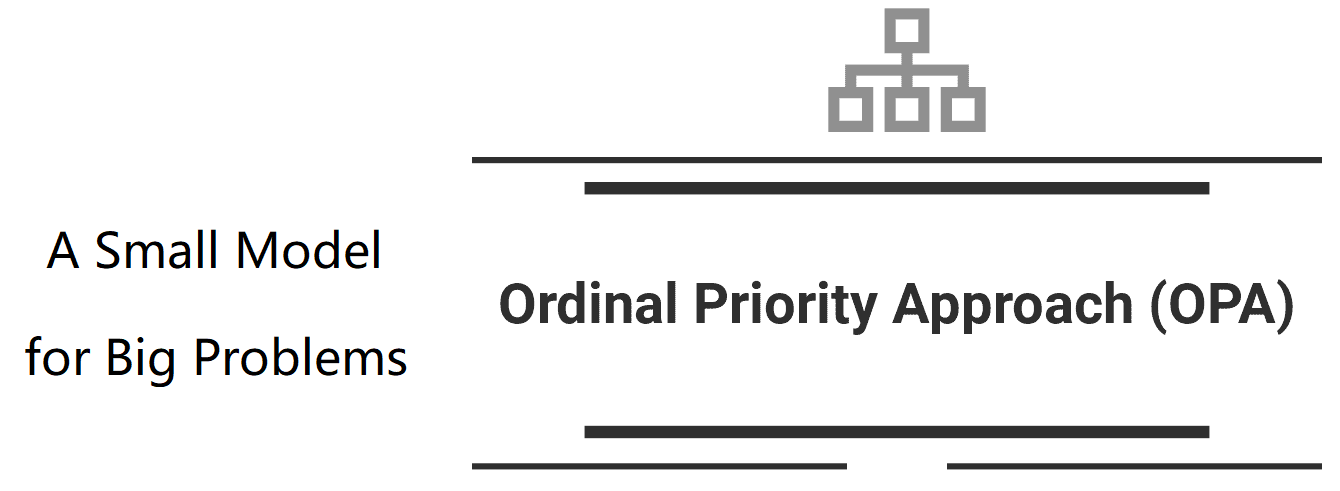Author

### Solving an Example Using the OPA

Here, we are going to solve the first example from Ordinal Priority Approach (OPA) in Multiple Attribute Decision-Making for the scholars who want to employ the OPA in their papers. This example is related to purchasing a car by considering the following attributes: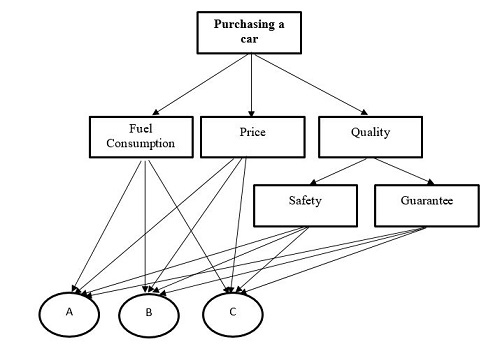It should be noted that there are three alternatives entitled A, B, and C. After identifying the attributes and alternatives, we should collect the data from the expert. The input data for this example can be seen in the following table:As can be seen from the above table, the expert declared that the order of the attributes is P > S > G > F. Moreover, the order of the alternatives in each attribute was provided. For example in attribute P, we have A > C > B. After collecting data, the OPA model should be constructed as follows: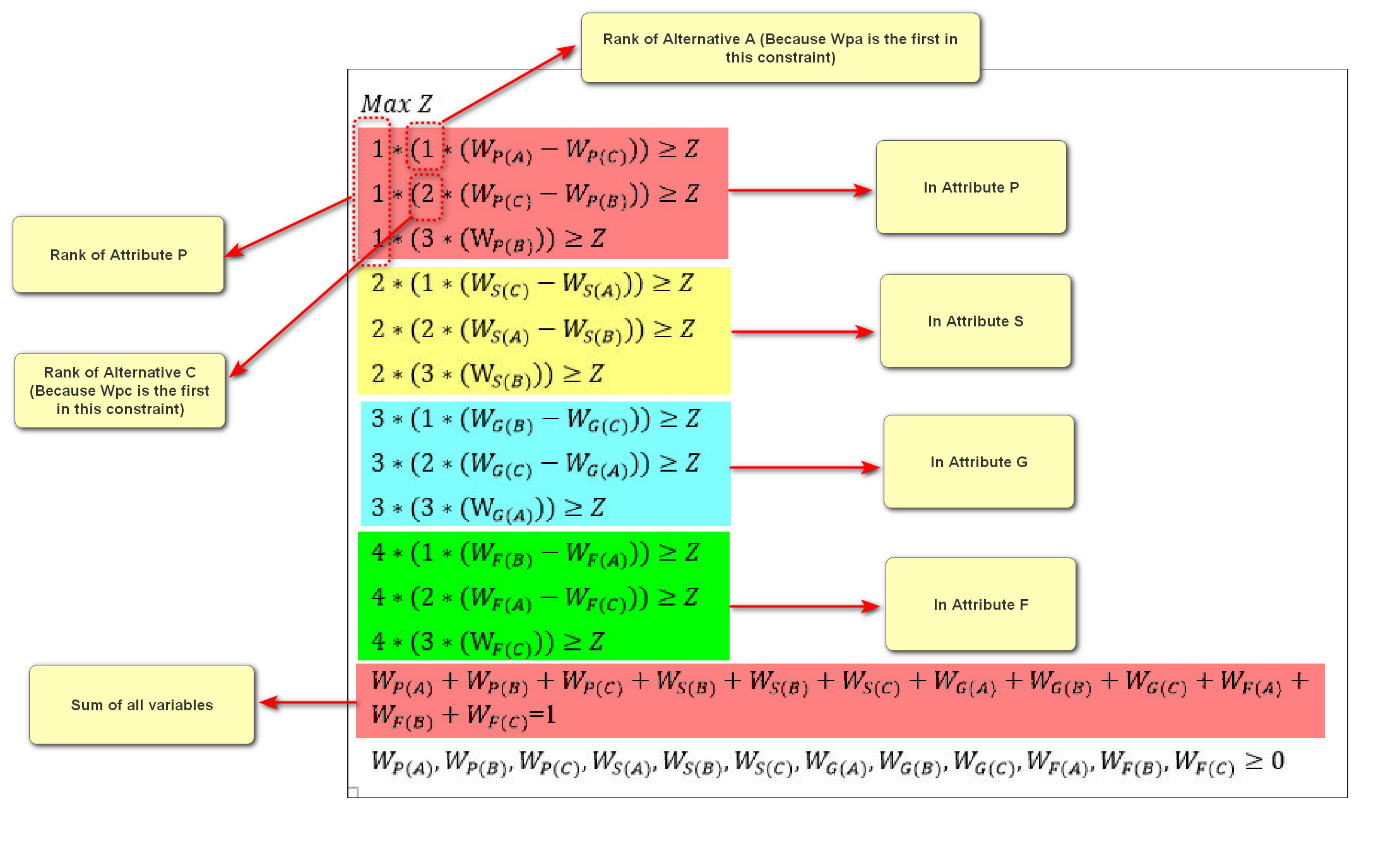This is a linear model which can be solved using various softwares such as GAMS, LINGO, Excel, etc. Then, the weight of the attributes can be calculated as follows: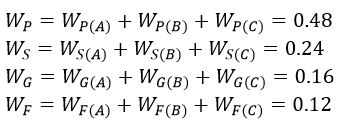Likewise, the weight of the alternatives can be calculated as follows: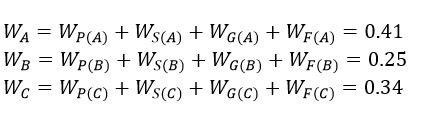Author